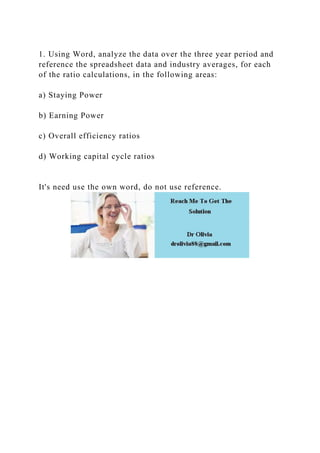Seu SlideShare está sendo baixado. ×

# 1. Using Word, analyze the data over the three year period and refer.docx

Anúncio
Anúncio
Anúncio
Anúncio
Anúncio
Anúncio
Anúncio
Anúncio
Anúncio
Anúncio
AnúncioCarregando em…3
×

1 de 1 Anúncio

# 1. Using Word, analyze the data over the three year period and refer.docx

1. Using Word, analyze the data over the three year period and reference the spreadsheet data and industry averages, for each of the ratio calculations, in the following areas:

a) Staying Power

b) Earning Power

c) Overall efficiency ratios

d) Working capital cycle ratios

It's need use the own word, do not use reference.
.

1. Using Word, analyze the data over the three year period and reference the spreadsheet data and industry averages, for each of the ratio calculations, in the following areas:

a) Staying Power

b) Earning Power

c) Overall efficiency ratios

d) Working capital cycle ratios

It's need use the own word, do not use reference.
.

Anúncio
Anúncio

### 1. Using Word, analyze the data over the three year period and refer.docx

1. 1. 1. Using Word, analyze the data over the three year period and reference the spreadsheet data and industry averages, for each of the ratio calculations, in the following areas: a) Staying Power b) Earning Power c) Overall efficiency ratios d) Working capital cycle ratios It's need use the own word, do not use reference.